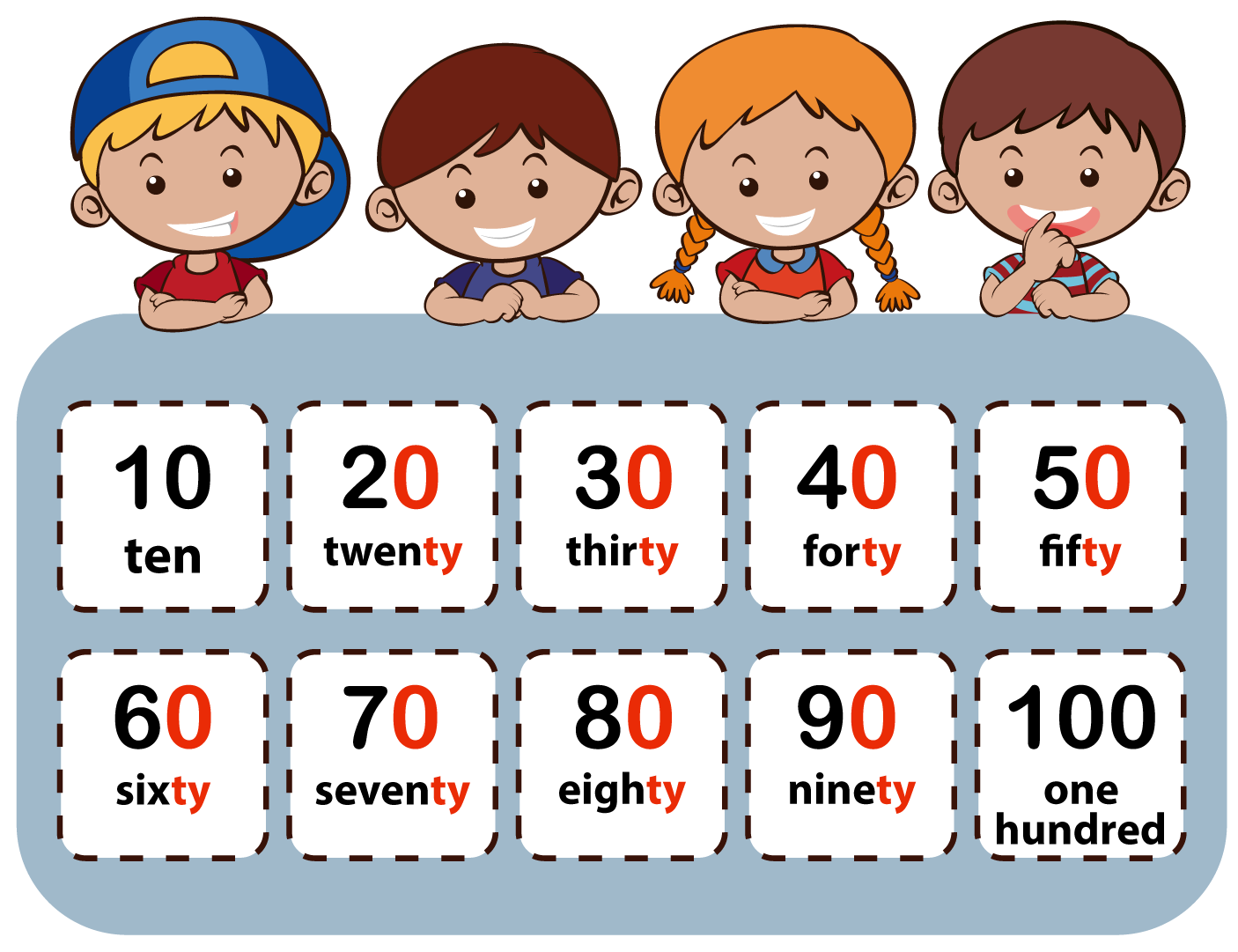Counting to 100 might sound a bit tricky, but it’s actually quite easy!

First, let’s look at numbers 1 to 10:

• 1 = one
• 2 = two
• 3 = three
• 4 = four
• 5 = five
• 6 = six
• 7 = seven
• 8 = eight
• 9 = nine
• 10 = ten

After number 10 there are two special numbers. These are different from other numbers:

• 11 = eleven
• 12 = twelve

The teen numbers come next. Their spelling always ends in teen and they go from 13 to 19:

• 13 = thirteen
• 14 = fourteen
• 15 = fifteen
• 16 = sixteen
• 17 = seventeen
• 18 = eighteen
• 19 = nineteen

What number comes after 19? It’s 20: twenty

Numbers that end with 0 end in ty, except 10 (ten) and 100 (one hundred).